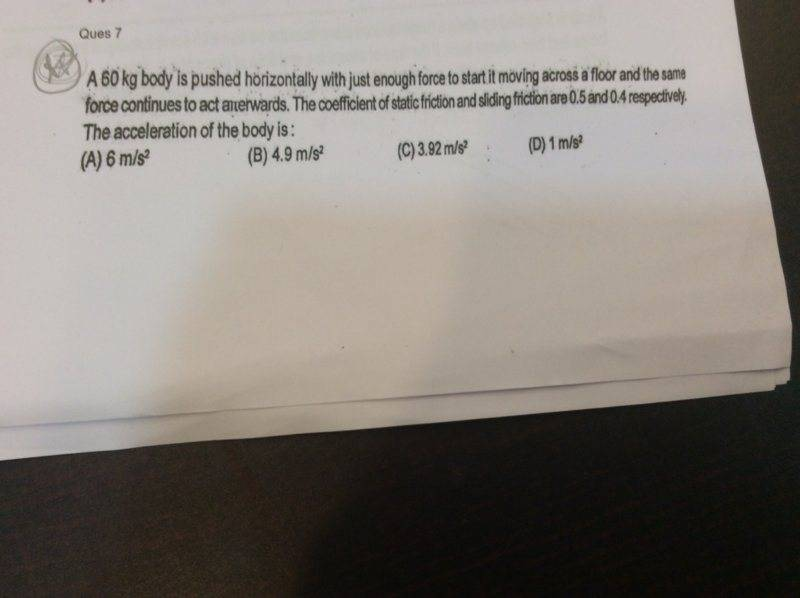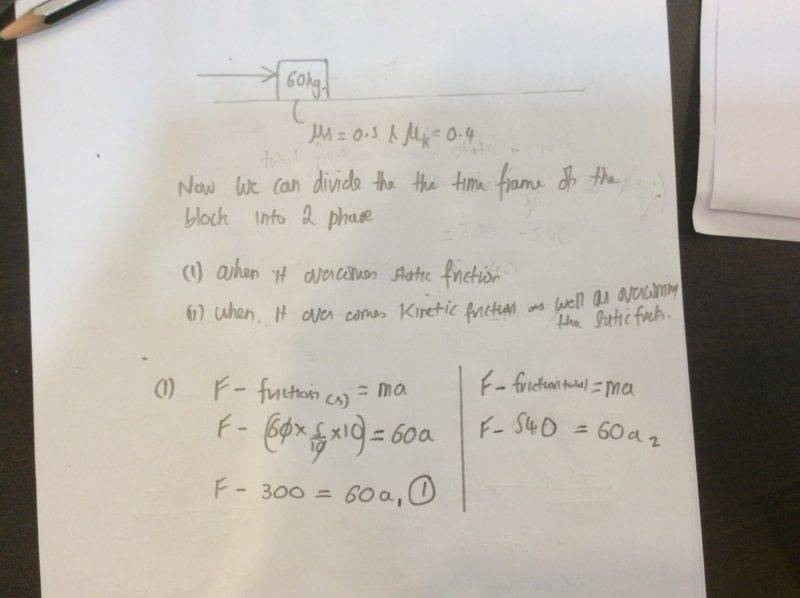# Finding the Accelation Of A Body In Presence Of Frictions1. Homework Statement

A 60 kg block is pushed horizontally with just enough force to start its motion Accross a floor and the same force acts on it afterwards.The coefficient of static and sliding frictions are 0.5 and 0.4 respectively.
Find acceleration of the body.
Options

6m/s^2

4.9m/s^2

3.92m/s^2

1m/s^2

## Homework Equations

F= ma

Friction = (u)m.a where " u " is coefficient of friction

## The Attempt at a Solution

First I assumed that the total force need would be the summation of all the friction forces acting on the body

So I did
m.a = friction static + friction kinetic

60. a = 540

a = 9 m/s^2
But alas tis the wrong answer.

Then I tried another method which is in the attachment above I hope it is visible. ....but I'm pretty sure it's wrong.

[So don't delete the post oh ,moderator supreme overlord for I have tried !!!]

I would really like to know how to approach this problem

THIS IS ALL THE INFO GIVEN

#### Attachments

Orodruin
Staff Emeritus
Homework Helper
Gold Member
2021 Award
The static coefficient of friction gives you the maximal possible friction force when the object is stationary. The kinetic coefficient of friction gives you the friction when the object is moving. They will not both be relevant at the same time.

The static coefficient of friction gives you the maximal possible friction force when the object is stationary. The kinetic coefficient of friction gives you the friction when the object is moving. They will not both be relevant at the same time.
So when I make the equation for in motion I should just consider kinetic right ?

and secandly since the force is constant will there be change in accelaration to that of when it was set into motion ?

Orodruin
Staff Emeritus
Homework Helper
Gold Member
2021 Award
Think about how you will use each piece of information. (Although there is one piece of information that is superfluous, but let's find out which. The first statement is that the force is just sufficient to get the body moving in the first place. What does this tell you about the force with which you are pushing the body?

Think about how you will use each piece of information. (Although there is one piece of information that is superfluous, but let's find out which. The first statement is that the force is just sufficient to get the body moving in the first place. What does this tell you about the force with which you are pushing the body?

Well i think it tells us that the force is just sufficient to tip the balance.like its at the Citical value...therefore I think it's right to assume(please correct me if I'm wrong)
That the force will be equal to static friction
Hence
F=Friction (static)
F = 300

Orodruin
Staff Emeritus
Homework Helper
Gold Member
2021 Award
That the force will be equal to static friction
Yes, this is correct. The force is just sufficient to overcome static friction, which in effect is saying that it is essentially equal to the maximal static friction.

Hence
F=Friction (static)
F = 300
Units, please! Units are important when you do physics. If you do not use them, you will have a difficult time. Even better in this case would be to leave the symbolic expression for the force and only input numbers at the very end. This is often (very) helpful in understanding and finding errors. In this case, what is the symbolic expression for the force ##F## expressed in the mass ##m##, the gravitational acceleration ##g##, and the coefficient of static friction ##\mu_s##?
(If you are unfamiliar with LaTeX, you can use the symbols available when you press the ##\Sigma## in the editor to get μ and the ##x_2## symbol to make a subindex s, all in all giving μs.)

↔↔
Yes, this is correct. The force is just sufficient to overcome static friction, which in effect is saying that it is essentially equal to the maximal static friction.

Units, please! Units are important when you do physics. If you do not use them, you will have a difficult time. Even better in this case would be to leave the symbolic expression for the force and only input numbers at the very end. This is often (very) helpful in understanding and finding errors. In this case, what is the symbolic expression for the force ##F## expressed in the mass ##m##, the gravitational acceleration ##g##, and the coefficient of static friction ##\mu_s##?
(If you are unfamiliar with LaTeX, you can use the symbols available when you press the ##\Sigma## in the editor to get μ and the ##x_2## symbol to make a subindex s, all in all giving μs.)
Great !!! I think I got the answer (hopefully) the working is in the attachment

I'm sorry for not using units,I'll make sure to use them henceforth.im extremely unfamiliar with the keyboard layout !!!

#### Attachments

Orodruin
Staff Emeritus
Homework Helper
Gold Member
2021 Award

Also, if you used symbolic expressions all the way rather than inserting numbers in every step, you would realise which piece of information in the problem was superfluous.

Also, if you used symbolic expressions all the way rather than inserting numbers in every step, you would realise which piece of information in the problem was superfluous.
Gosh I should really read the terms and conditions !!! Sorry for the attachments

mass is superfluous isn't it ?

Orodruin
Staff Emeritus
Homework Helper
Gold Member
2021 Award
mass is superfluous isn't it ?
Yes. All of the forces are proportional to the mass, which means it cancels out with the ##m## in Newton's second law.

Yes. All of the forces are proportional to the mass, which means it cancels out with the ##m## in Newton's second law.
Thank you so much ! This problem has been bugging me for a while .

Anyway have an awesome day !!!!